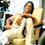# Amazing function (Collatz Problem)

$f\left( x \right) =\quad \quad \quad 3x+1\quad \quad \forall \quad x\quad \varepsilon \quad odd\quad positive\quad integer\\ \quad \quad \quad =\quad \quad \quad x/2\quad \quad \quad \quad \forall \quad x\quad \varepsilon \quad even\quad positive\quad integer$

use this function repeatedly you will always get the answer as 1( it may take a numerous steps for certain numbers ) it always ends with the series 4,2,1

let x=28

Operating this function repeatedly (Used the function for 11 times)

x=28>14>7>22>11>34>17>52>26>13>40>20>10>5>16>8>4>2>1

let x=37

Operating this function repeatedly (used the function for 71 times)

x=37>111>334>167>502>251>754>377>1132>566>283>850>425>1276>638>319>958>479>1438>719>2158

1079>3238>1619>4858>2429>7288>3644>1822>911>2734>1367>4102>2051>6154>3077>9232>4616>2308>1154>577>1732>866>433>1300>650>325>976>488>244>122>61>184>92>46>23>70>35>106>53>160>80>40>20>10>5>16>8>4>2>1Note by Anirudha Nayak
7 years, 3 months ago

This discussion board is a place to discuss our Daily Challenges and the math and science related to those challenges. Explanations are more than just a solution — they should explain the steps and thinking strategies that you used to obtain the solution. Comments should further the discussion of math and science.

When posting on Brilliant:

• Use the emojis to react to an explanation, whether you're congratulating a job well done , or just really confused .
• Ask specific questions about the challenge or the steps in somebody's explanation. Well-posed questions can add a lot to the discussion, but posting "I don't understand!" doesn't help anyone.
• Try to contribute something new to the discussion, whether it is an extension, generalization or other idea related to the challenge.

MarkdownAppears as
*italics* or _italics_ italics
**bold** or __bold__ bold
- bulleted- list
• bulleted
• list
1. numbered2. list
1. numbered
2. list
Note: you must add a full line of space before and after lists for them to show up correctly
paragraph 1paragraph 2

paragraph 1

paragraph 2

[example link](https://brilliant.org)example link
> This is a quote
This is a quote
    # I indented these lines
# 4 spaces, and now they show
# up as a code block.

print "hello world"
# I indented these lines
# 4 spaces, and now they show
# up as a code block.

print "hello world"
MathAppears as
Remember to wrap math in $$ ... $$ or $ ... $ to ensure proper formatting.
2 \times 3 $2 \times 3$
2^{34} $2^{34}$
a_{i-1} $a_{i-1}$
\frac{2}{3} $\frac{2}{3}$
\sqrt{2} $\sqrt{2}$
\sum_{i=1}^3 $\sum_{i=1}^3$
\sin \theta $\sin \theta$
\boxed{123} $\boxed{123}$

Sort by:

Nice function, but there is a mistake when you put $x=28$. When plugging in $x=11$, you get $34$ and not $32$.

- 7 years, 3 months ago

It is an open conjecture that it "always ends with the series 4, 2, 1". It's been verified for the first few cases, but there are infinitely many more possibilities left.

Staff - 7 years, 3 months ago